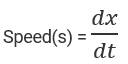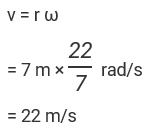# Linear Speed Formula

## Linear Speed Formulas Physics

Linear speed is defined as rate of change of distance or distance covered in unit time.
If, dx is change in distance and dt is the change in speed, then linear speed is given by:SI unit of linear speed is m/s.
The relation between linear speed(x) and angular speed(ω) in case of circular motion is given by:
Speed(s) =
Where, r = radius of the circular path

Example:
Calculate the linear speed of a body moving at 30 rpm in a circular path having a radius of 7 m?